# The Normal Distribution Central Limit Theorem and Introduction

• Slides: 97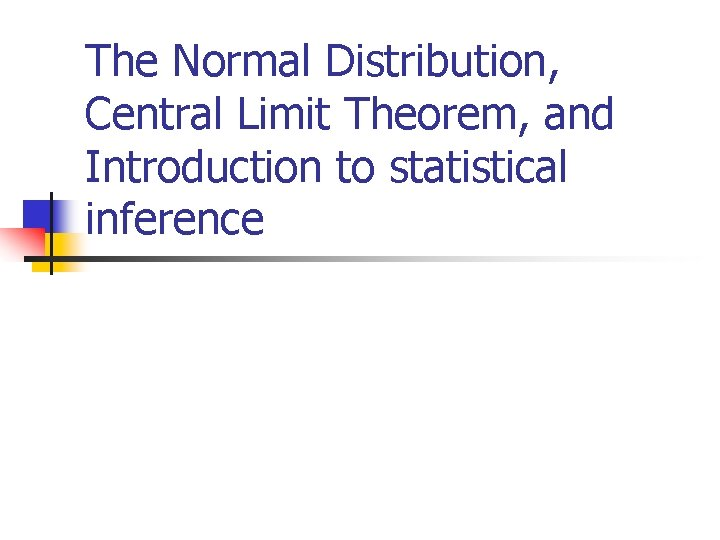The Normal Distribution, Central Limit Theorem, and Introduction to statistical inference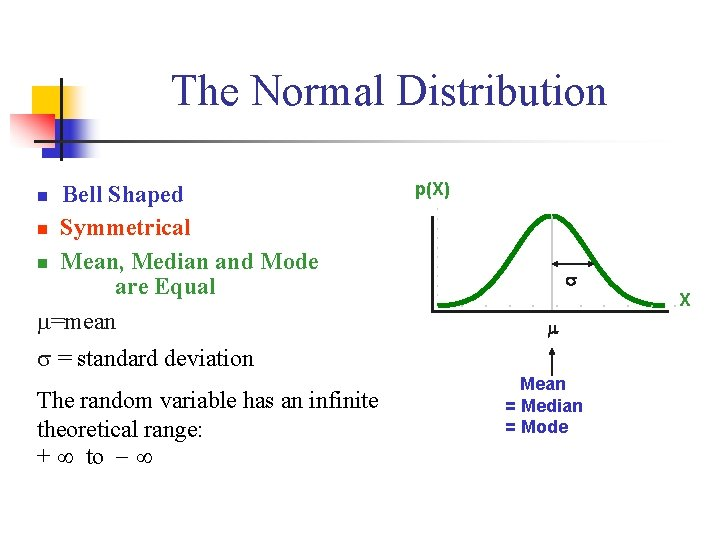The Normal Distribution n ‘Bell Shaped’ n Symmetrical n Mean, Median and Mode are Equal =mean p(X) s X m = standard deviation The random variable has an infinite theoretical range: + to Mean = Median = Mode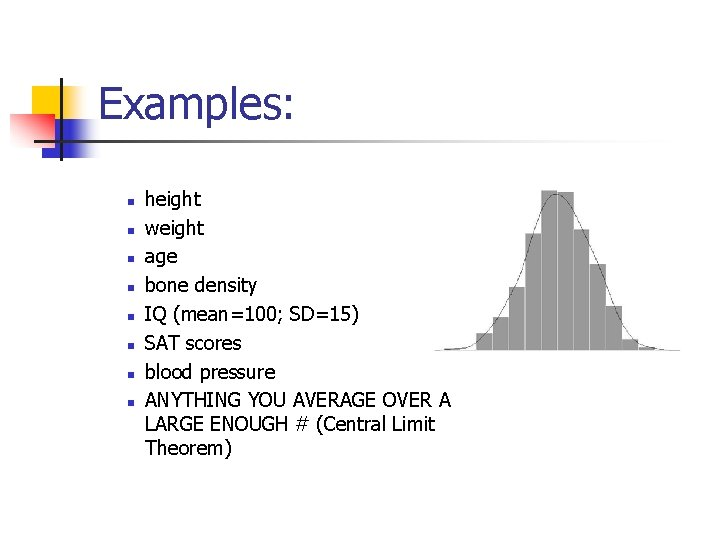Examples: n n n n height weight age bone density IQ (mean=100; SD=15) SAT scores blood pressure ANYTHING YOU AVERAGE OVER A LARGE ENOUGH # (Central Limit Theorem)Small standard deviation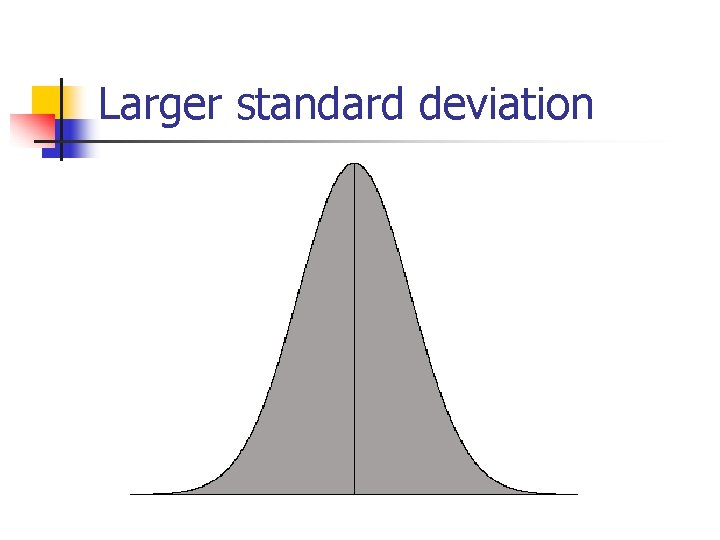Larger standard deviationEven larger standard deviationThe Normal Distribution: as mathematical function (pdf) Note constants: =3. 14159 e=2. 71828 This is a bell shaped curve with different centers and spreads depending on and68 -95 -99. 7 Rule in Math terms…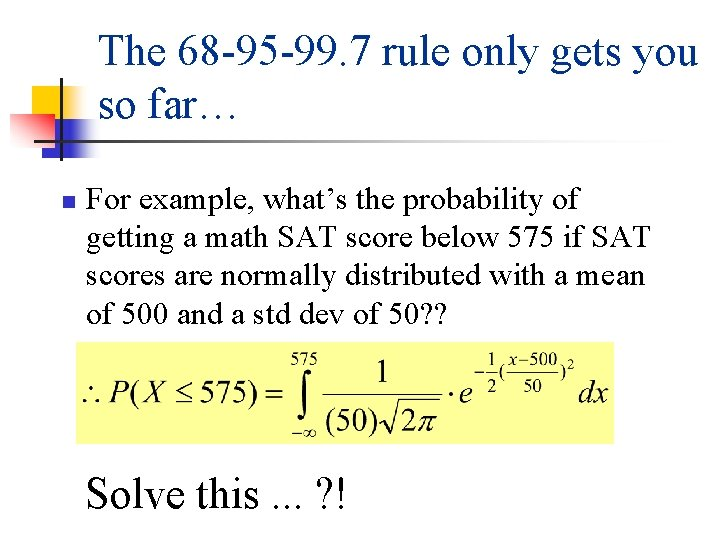The 68 -95 -99. 7 rule only gets you so far… n For example, what’s the probability of getting a math SAT score below 575 if SAT scores are normally distributed with a mean of 500 and a std dev of 50? ? Solve this. . . ? !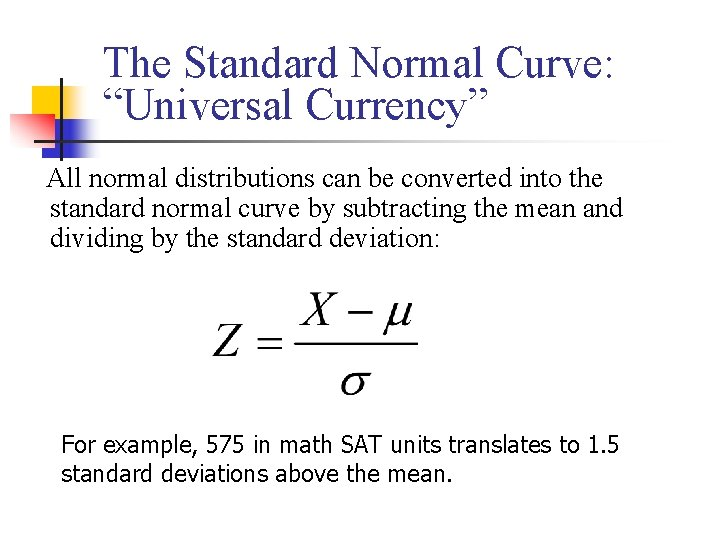The Standard Normal Curve: “Universal Currency” All normal distributions can be converted into the standard normal curve by subtracting the mean and dividing by the standard deviation: For example, 575 in math SAT units translates to 1. 5 standard deviations above the mean.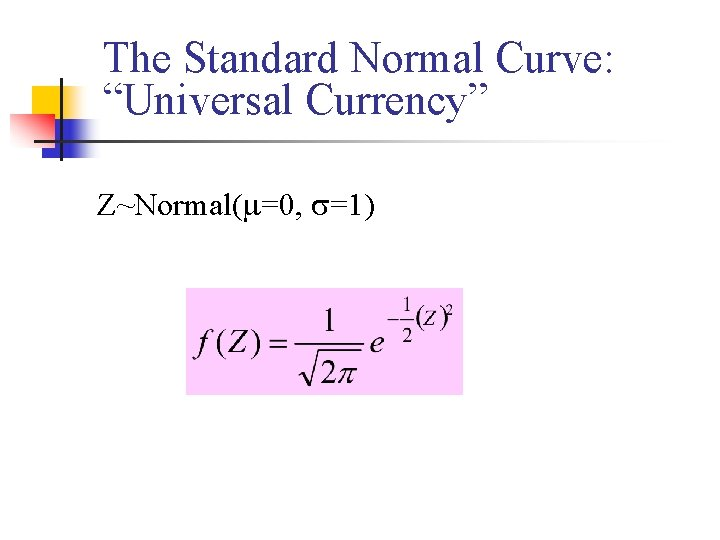The Standard Normal Curve: “Universal Currency” Z~Normal( =0, =1)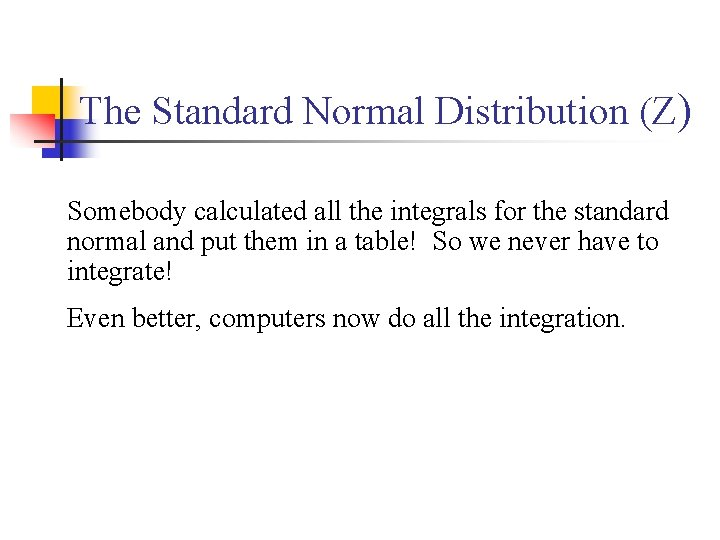The Standard Normal Distribution (Z) Somebody calculated all the integrals for the standard normal and put them in a table! So we never have to integrate! Even better, computers now do all the integration.Comparing X and Z units 500 0 575 1. 5 X Z ( = 500, = 50) ( = 0, = 1)Example So, What’s the probability of getting a math SAT score of 575 or less, =500 and =50? No need to do the integration! Just look up Z= 1. 5 in standard normal chart no problem! =. 9332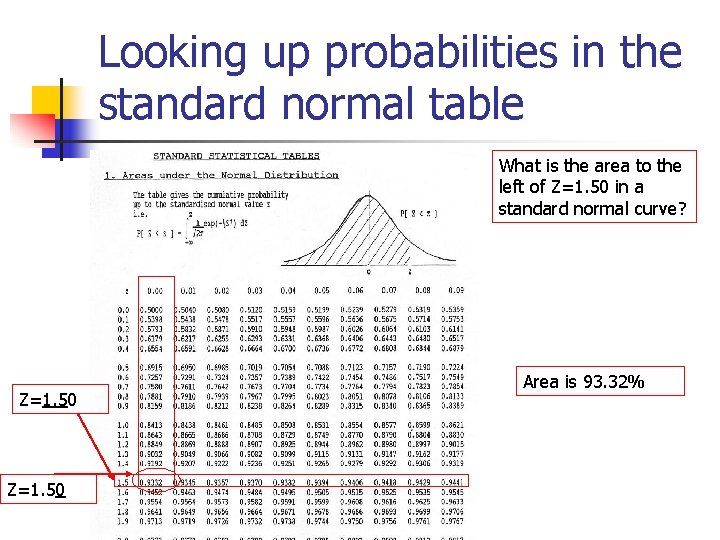Looking up probabilities in the standard normal table What is the area to the left of Z=1. 50 in a standard normal curve? Z=1. 50 Area is 93. 32%Exercise (in groups of 2 -3) a. b. If birth weights in a population are normally distributed with a mean of 109 oz and a standard deviation of 13 oz, What is the chance of obtaining a birth weight of 141 oz or heavier when sampling birth records at random? What is the chance of obtaining a birth weight of 120 or lighter?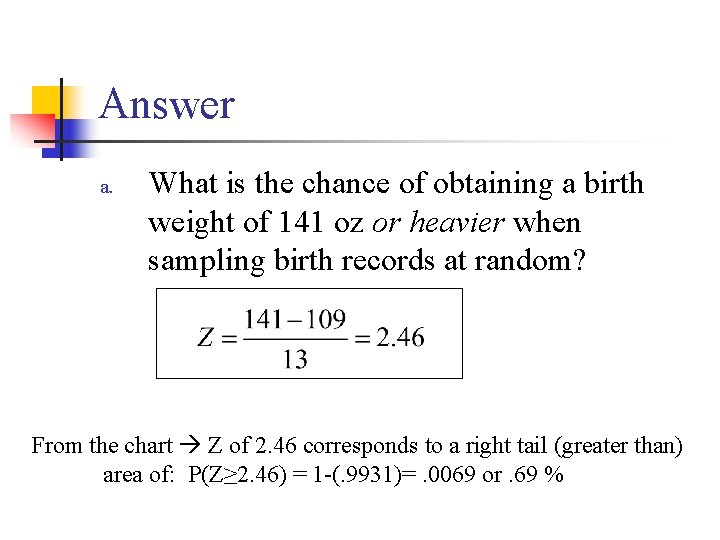Answer a. What is the chance of obtaining a birth weight of 141 oz or heavier when sampling birth records at random? From the chart Z of 2. 46 corresponds to a right tail (greater than) area of: P(Z≥ 2. 46) = 1 -(. 9931)=. 0069 or. 69 %Answer b. What is the chance of obtaining a birth weight of 120 or lighter? From the chart Z of. 85 corresponds to a left tail area of: P(Z≤. 85) =. 8023= 80. 23%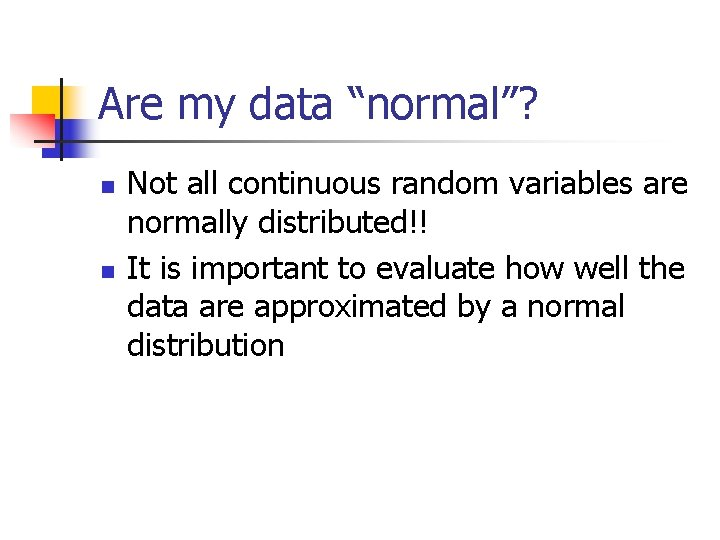Are my data “normal”? n n Not all continuous random variables are normally distributed!! It is important to evaluate how well the data are approximated by a normal distribution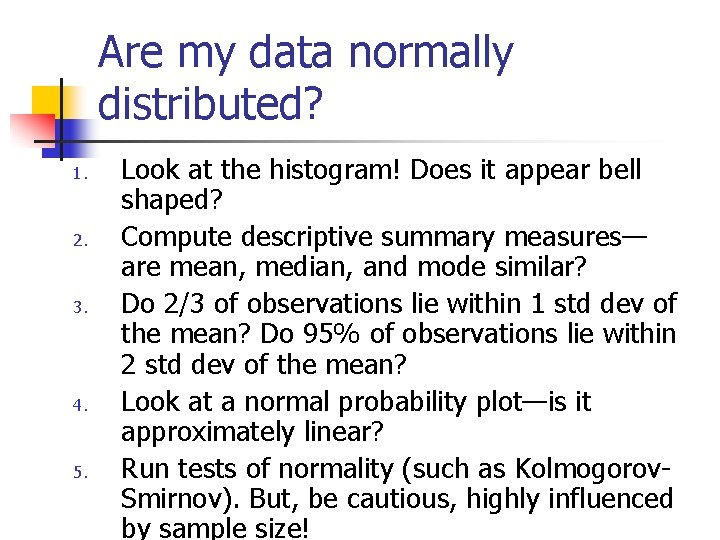Are my data normally distributed? 1. 2. 3. 4. 5. Look at the histogram! Does it appear bell shaped? Compute descriptive summary measures— are mean, median, and mode similar? Do 2/3 of observations lie within 1 std dev of the mean? Do 95% of observations lie within 2 std dev of the mean? Look at a normal probability plot—is it approximately linear? Run tests of normality (such as Kolmogorov. Smirnov). But, be cautious, highly influenced by sample size!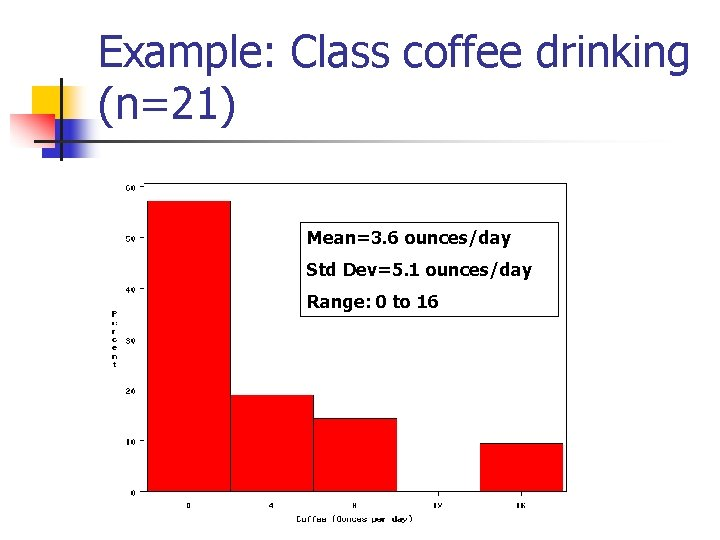Example: Class coffee drinking (n=21) Mean=3. 6 ounces/day Std Dev=5. 1 ounces/day Range: 0 to 16Example: Class coffee drinking (n=21) Mean 1 Std Dev= 3. 6 5. 1 = -1. 5 to 8. 7 -1. 5 8. 7 (covers 90% of observations)Example: Class coffee drinking (n=21) Mean 2 Std Dev= 3. 6 10. 2 = -6. 6 to 13. 8 (covers 90% of observations) 13. 8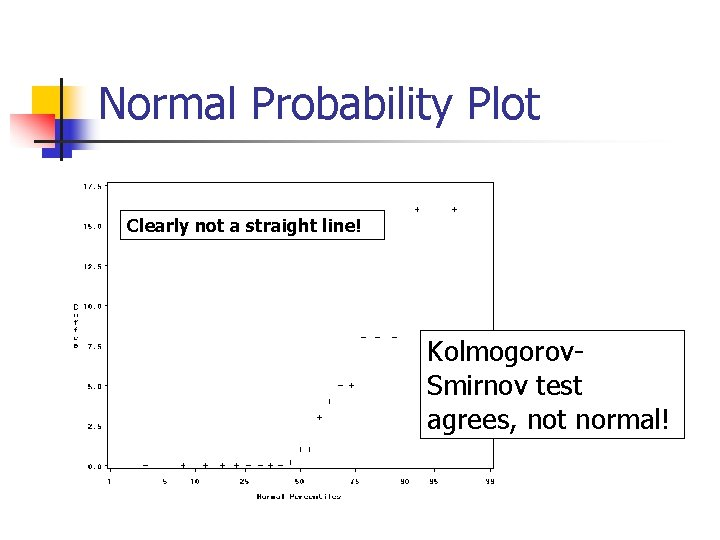Normal Probability Plot Clearly not a straight line! Kolmogorov. Smirnov test agrees, not normal!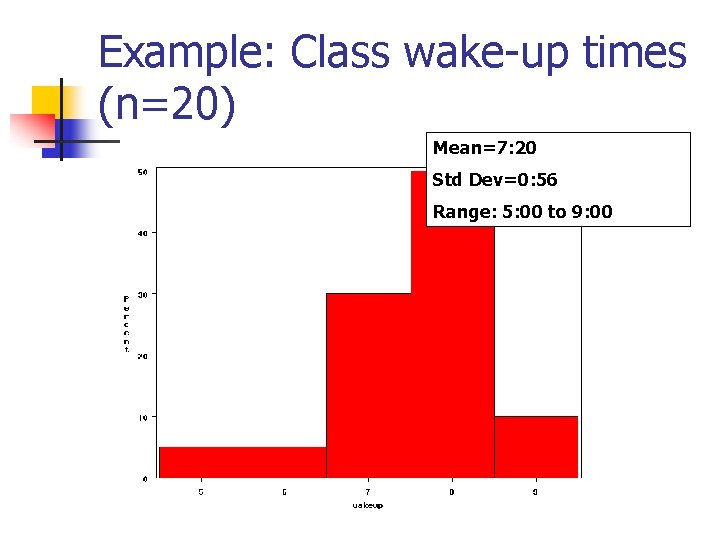Example: Class wake-up times (n=20) Mean=7: 20 Std Dev=0: 56 Range: 5: 00 to 9: 00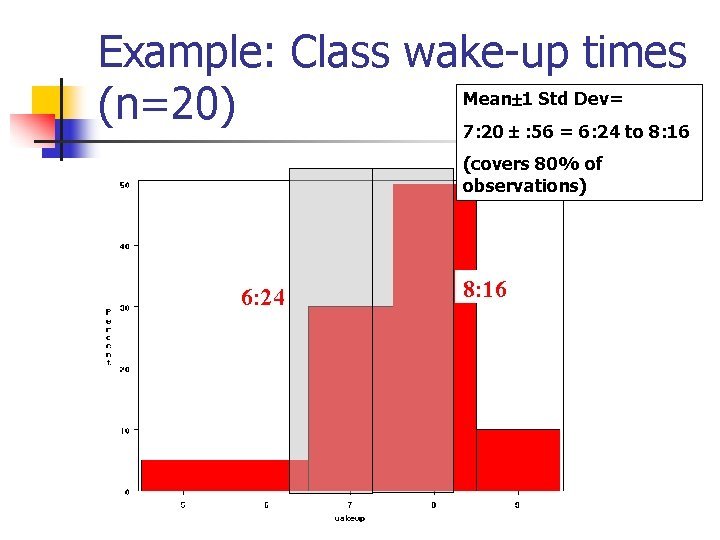Example: Class wake-up times Mean 1 Std Dev= (n=20) 7: 20 : 56 = 6: 24 to 8: 16 (covers 80% of observations) 6: 24 8: 16Example: Class wake-up times (n=20) Mean 2 Std Dev= 7: 20 1: 52 = 5: 28 to 9: 12 (covers 95% of observations)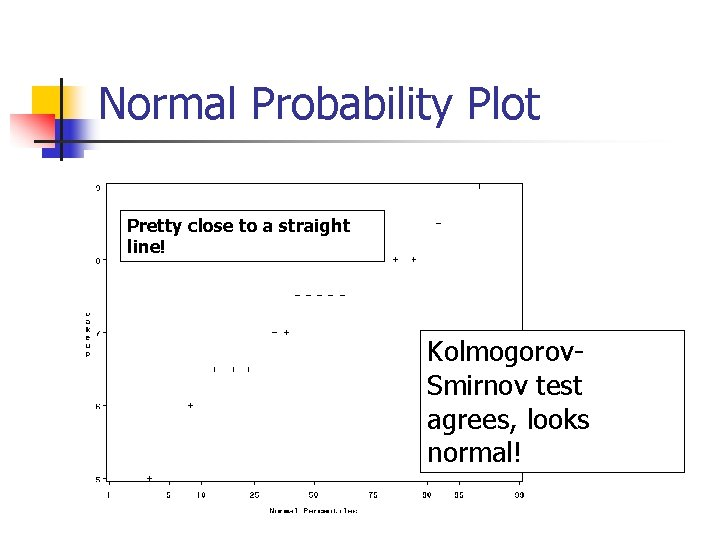Normal Probability Plot Pretty close to a straight line! Kolmogorov. Smirnov test agrees, looks normal!Review Problem 1 Which of the following about the normal distribution is NOT true? a. b. c. d. Theoretically, the mean, median, and mode are the same. About 2/3 of the observations fall within 1 standard deviation from the mean. It is a discrete probability distribution. Its parameters are the mean, , and standard deviation, .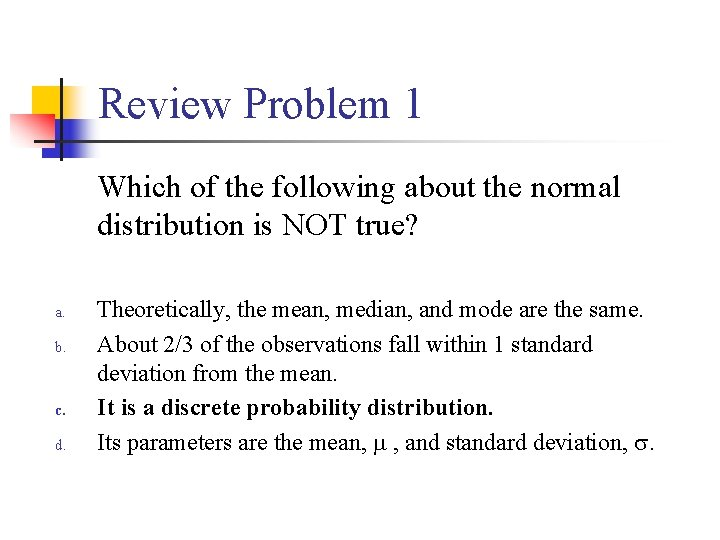Review Problem 1 Which of the following about the normal distribution is NOT true? a. b. c. d. Theoretically, the mean, median, and mode are the same. About 2/3 of the observations fall within 1 standard deviation from the mean. It is a discrete probability distribution. Its parameters are the mean, , and standard deviation, .Review Problem 2 For some positive value of Z, the probability that a standard normal variable is between 0 and Z is 0. 3770. The value of Z is: a. b. c. d. 0. 18. 0. 81. 1. 16 1. 47.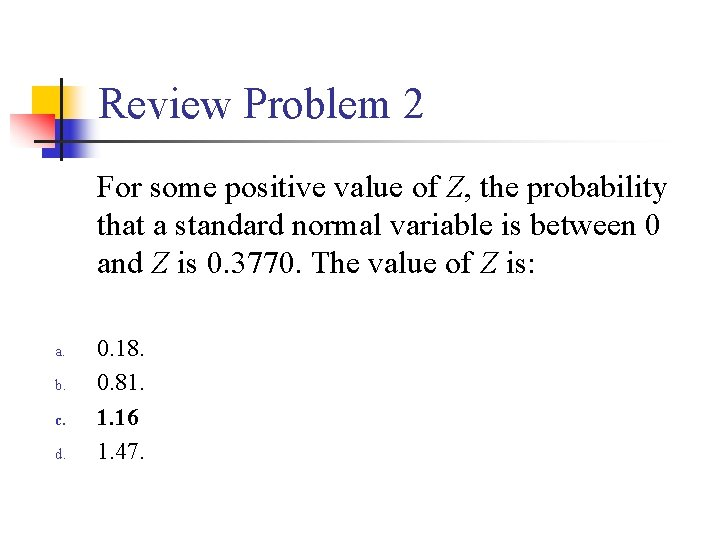Review Problem 2 For some positive value of Z, the probability that a standard normal variable is between 0 and Z is 0. 3770. The value of Z is: a. b. c. d. 0. 18. 0. 81. 1. 16 1. 47.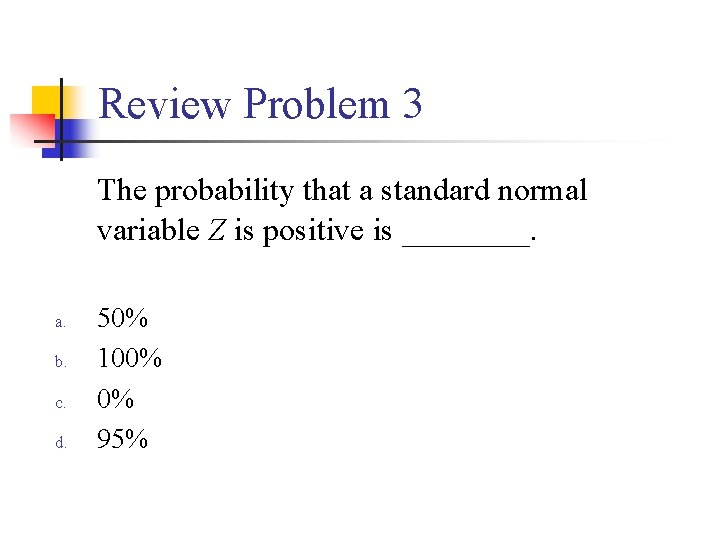Review Problem 3 The probability that a standard normal variable Z is positive is ____. a. b. c. d. 50% 100% 0% 95%Review Problem 3 The probability that a standard normal variable Z is positive is ____. a. b. c. d. 50% 100% 0% 95%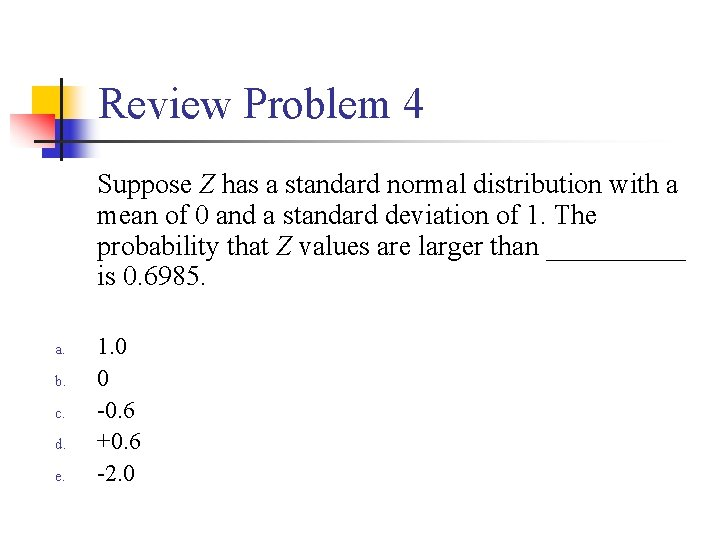Review Problem 4 Suppose Z has a standard normal distribution with a mean of 0 and a standard deviation of 1. The probability that Z values are larger than _____ is 0. 6985. a. b. c. d. e. 1. 0 0 -0. 6 +0. 6 -2. 0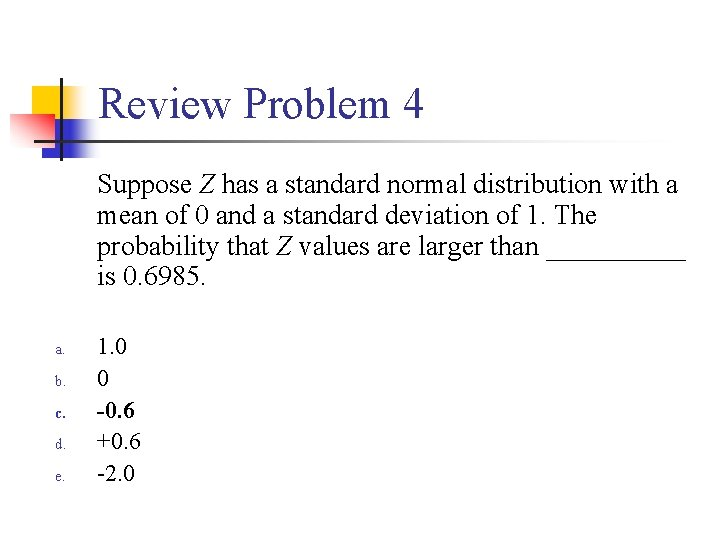Review Problem 4 Suppose Z has a standard normal distribution with a mean of 0 and a standard deviation of 1. The probability that Z values are larger than _____ is 0. 6985. a. b. c. d. e. 1. 0 0 -0. 6 +0. 6 -2. 0Statistical Inference: Hypothesis Testing and Confidence IntervalsWhat is a statistic? n n A statistic is any value that can be calculated from the sample data. Sample statistics are calculated to give us an idea about the larger population.Examples of statistics: n mean n n difference in means n n The difference in the average gas price in San Francisco (\$3. 37) compared with Minneapolis (\$2. 65) is 72 cents. proportion n n The average cost of a gallon of gas in the US is \$2. 87. 67% of high school students in the U. S. exercise regularly difference in proportions n The difference in the proportion of men who approve of George W. (44%) and women who do (38%) is 6%What is a statistic? n Sample statistics are estimates of population parameters.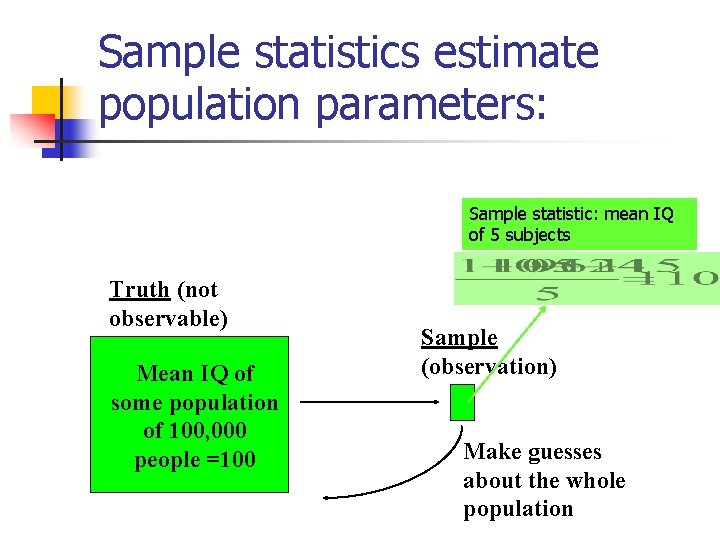Sample statistics estimate population parameters: Sample statistic: mean IQ of 5 subjects Truth (not observable) Mean IQ of some population of 100, 000 people =100 Sample (observation) Make guesses about the whole population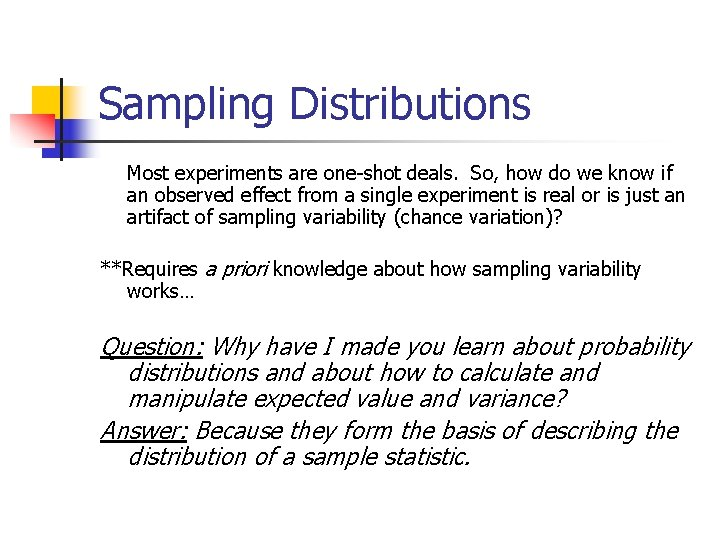Sampling Distributions Most experiments are one-shot deals. So, how do we know if an observed effect from a single experiment is real or is just an artifact of sampling variability (chance variation)? **Requires a priori knowledge about how sampling variability works… Question: Why have I made you learn about probability distributions and about how to calculate and manipulate expected value and variance? Answer: Because they form the basis of describing the distribution of a sample statistic.What is sampling variation? n n Statistics vary from sample to sample due to random chance. Example: A population of 100, 000 people has an average IQ of 100 (If you actually could measure them all!) If you sample 5 random people from this population, what will you get?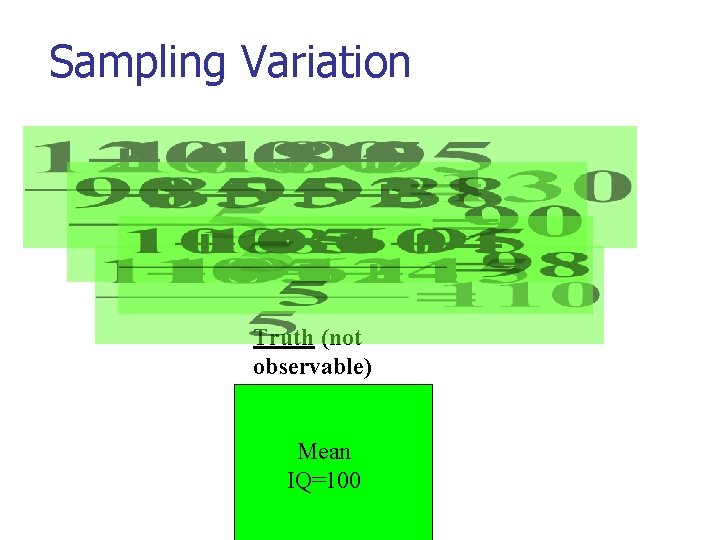Sampling Variation Truth (not observable) Mean IQ=100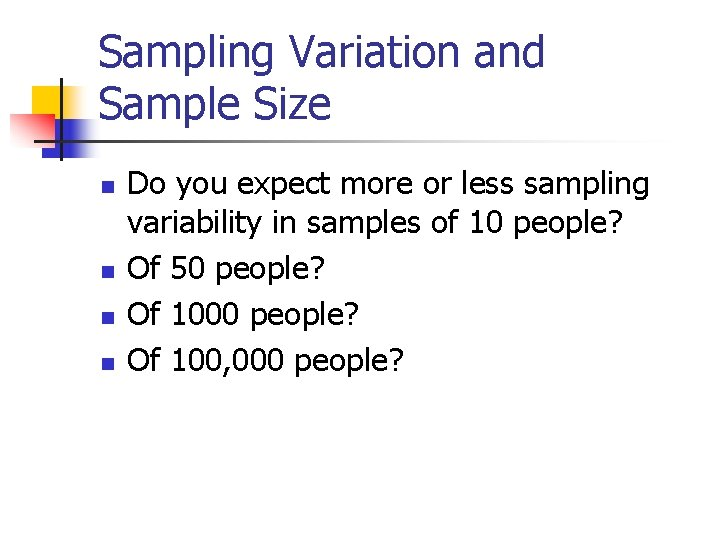Sampling Variation and Sample Size n n Do you expect more or less sampling variability in samples of 10 people? Of 50 people? Of 100, 000 people?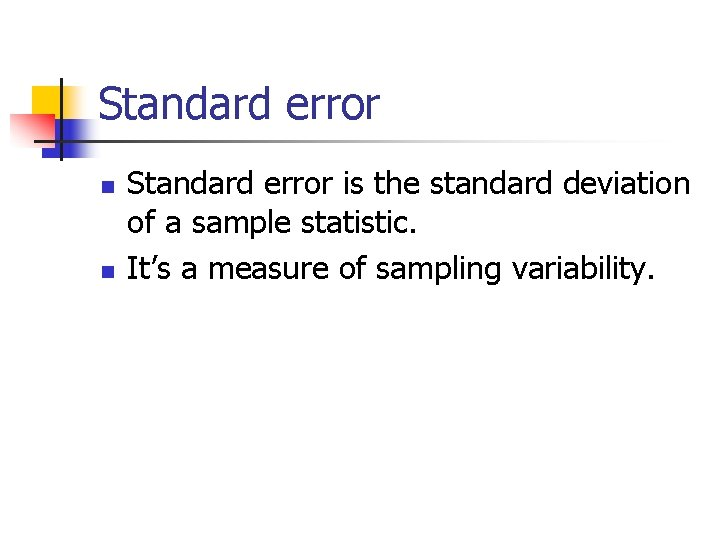Standard error n n Standard error is the standard deviation of a sample statistic. It’s a measure of sampling variability.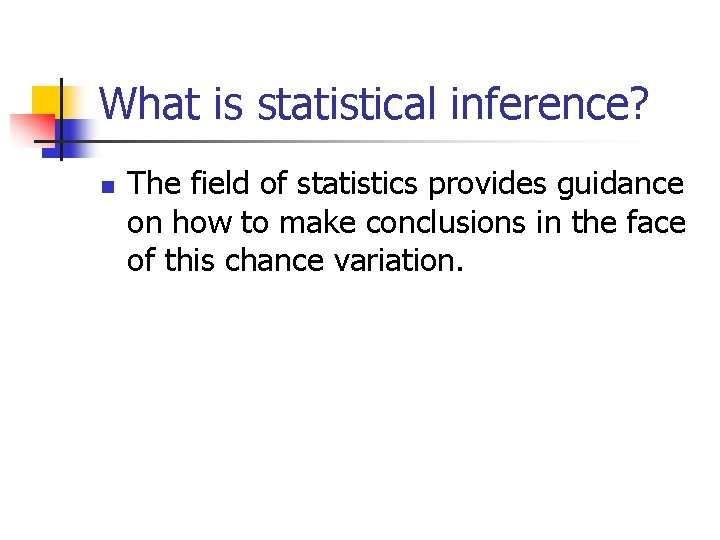What is statistical inference? n The field of statistics provides guidance on how to make conclusions in the face of this chance variation.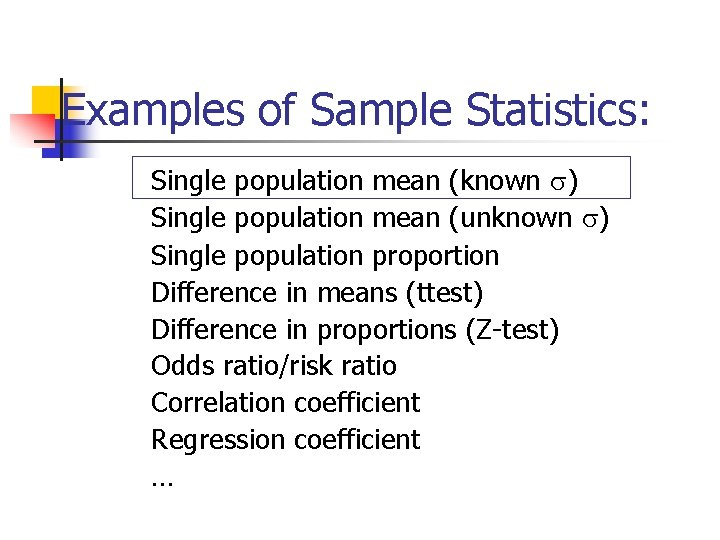Examples of Sample Statistics: Single population mean (known ) Single population mean (unknown ) Single population proportion Difference in means (ttest) Difference in proportions (Z-test) Odds ratio/risk ratio Correlation coefficient Regression coefficient …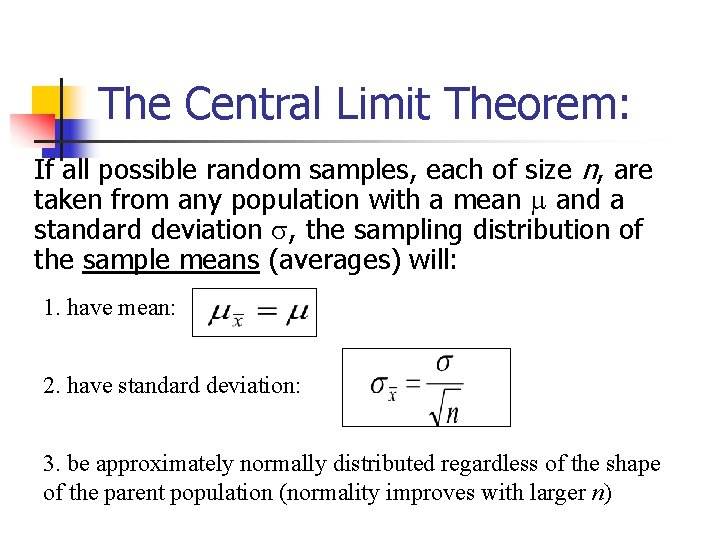The Central Limit Theorem: If all possible random samples, each of size n, are taken from any population with a mean and a standard deviation , the sampling distribution of the sample means (averages) will: 1. have mean: 2. have standard deviation: 3. be approximately normally distributed regardless of the shape of the parent population (normality improves with larger n)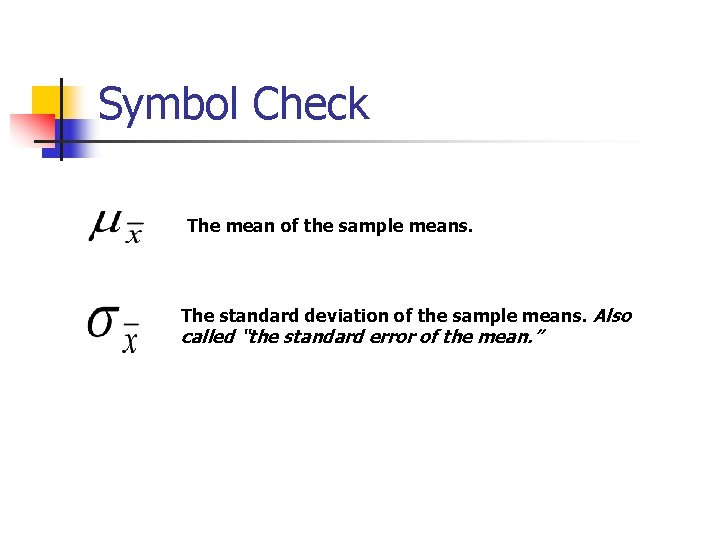Symbol Check The mean of the sample means. The standard deviation of the sample means. Also called “the standard error of the mean. ”Computer simulation of the sampling distribution of the sample mean: 1. Pick any probability distribution and specify a mean and standard deviation. 2. Tell the computer to randomly generate 1000 observations from that probability distributions E. g. , the computer is more likely to spit out values with high probabilities 3. Plot the “observed” values in a histogram. 4. Next, tell the computer to randomly generate 1000 averages-of -2 (randomly pick 2 and take their average) from that probability distribution. Plot “observed” averages in histograms. 5. Repeat for averages-of-10, and averages-of-100.Uniform on [0, 1]: average of 1 (original distribution)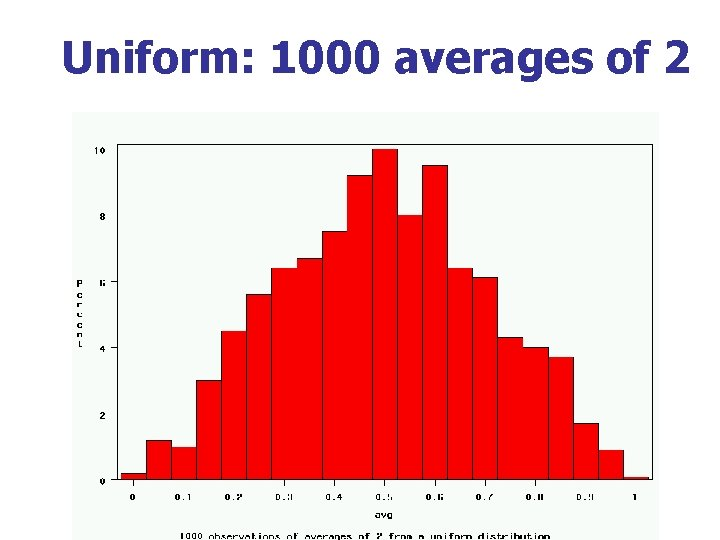Uniform: 1000 averages of 2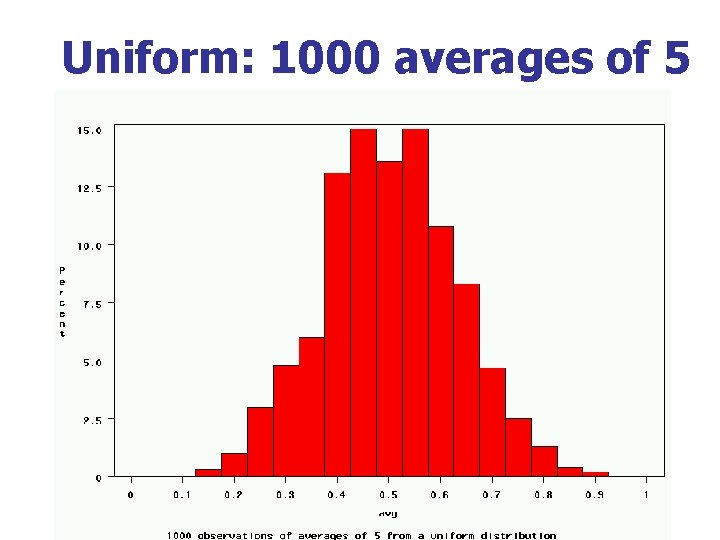Uniform: 1000 averages of 5Uniform: 1000 averages of 100~Exp(1): average of 1 (original distribution)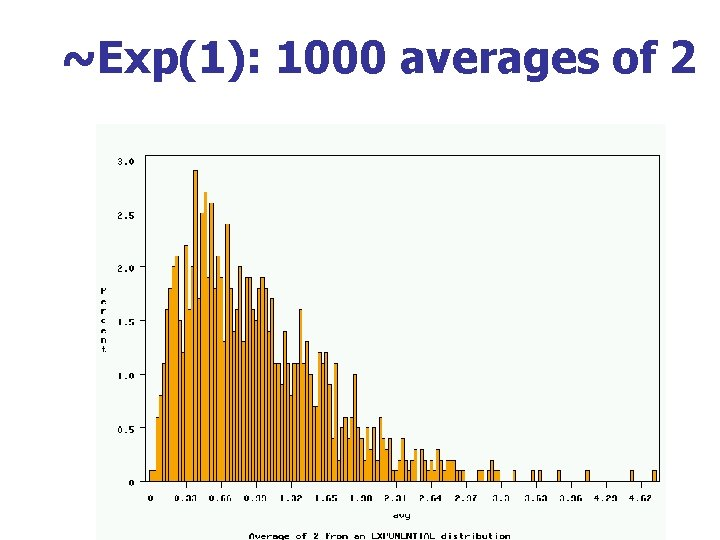~Exp(1): 1000 averages of 2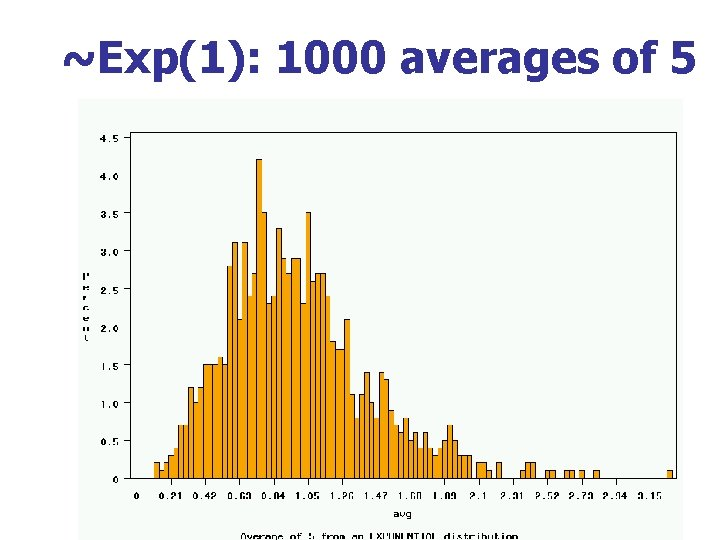~Exp(1): 1000 averages of 5~Exp(1): 1000 averages of 100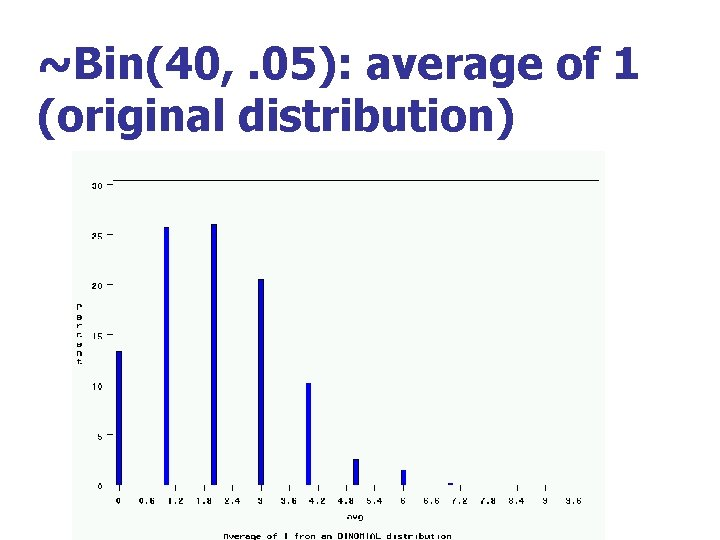~Bin(40, . 05): average of 1 (original distribution)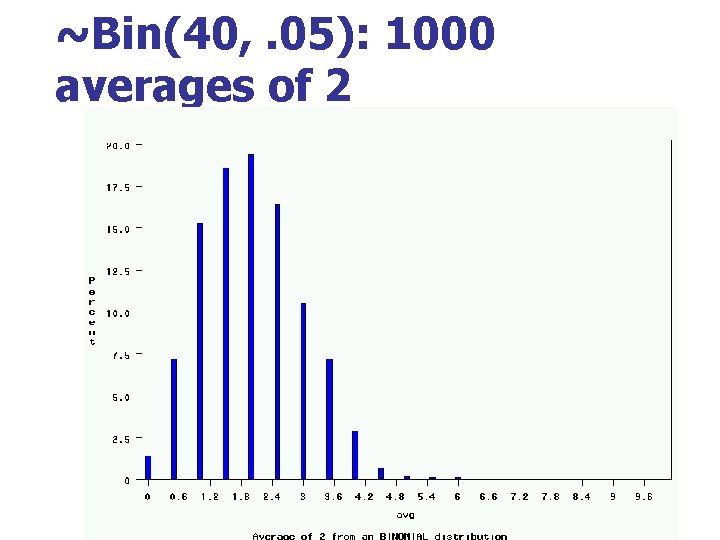~Bin(40, . 05): 1000 averages of 2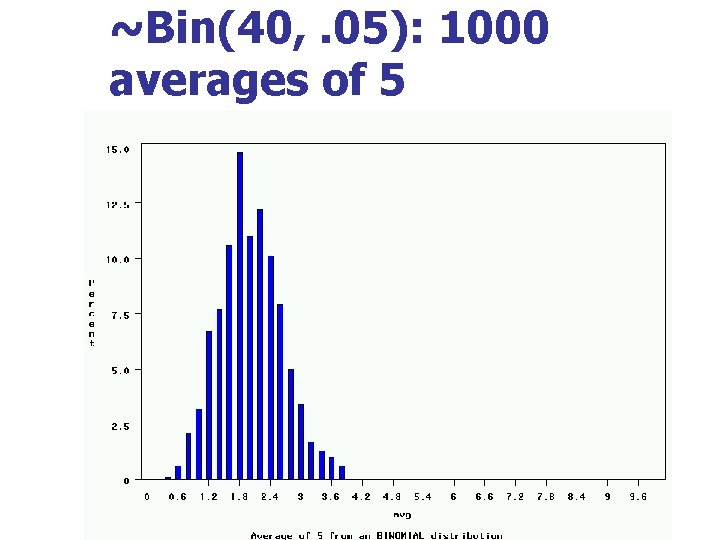~Bin(40, . 05): 1000 averages of 5~Bin(40, . 05): 1000 averages of 100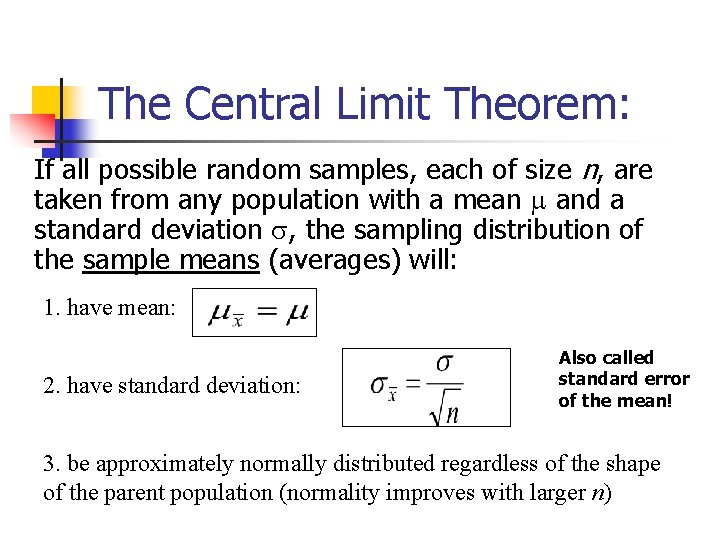The Central Limit Theorem: If all possible random samples, each of size n, are taken from any population with a mean and a standard deviation , the sampling distribution of the sample means (averages) will: 1. have mean: 2. have standard deviation: Also called standard error of the mean! 3. be approximately normally distributed regardless of the shape of the parent population (normality improves with larger n)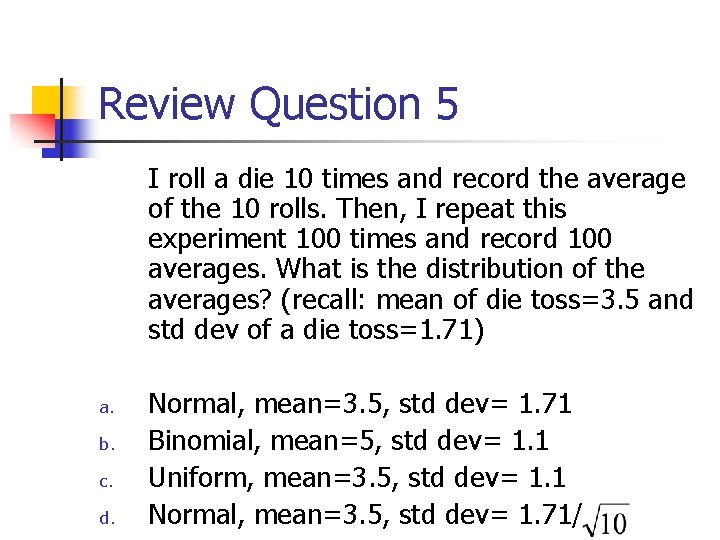Review Question 5 I roll a die 10 times and record the average of the 10 rolls. Then, I repeat this experiment 100 times and record 100 averages. What is the distribution of the averages? (recall: mean of die toss=3. 5 and std dev of a die toss=1. 71) a. b. c. d. Normal, mean=3. 5, std dev= 1. 71 Binomial, mean=5, std dev= 1. 1 Uniform, mean=3. 5, std dev= 1. 1 Normal, mean=3. 5, std dev= 1. 71/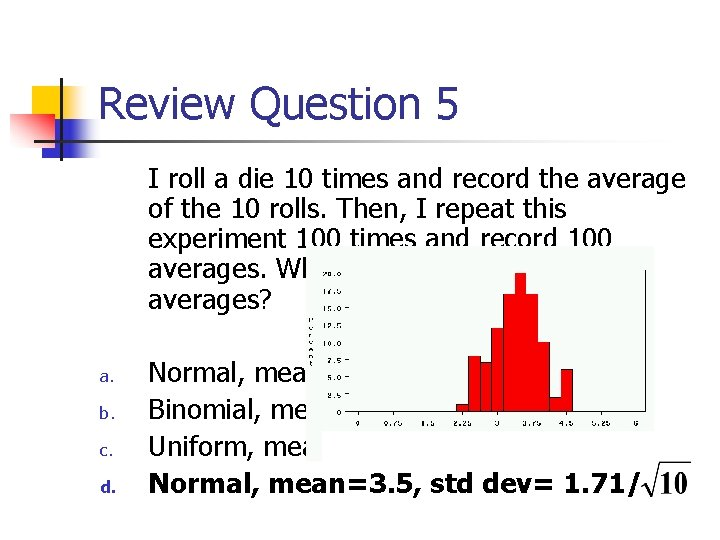Review Question 5 I roll a die 10 times and record the average of the 10 rolls. Then, I repeat this experiment 100 times and record 100 averages. What is the distribution of the averages? a. b. c. d. Normal, mean=3. 5, std dev= 1. 71 Binomial, mean=5, std dev= 1. 1 Uniform, mean=3. 5, std dev= 1. 1 Normal, mean=3. 5, std dev= 1. 71/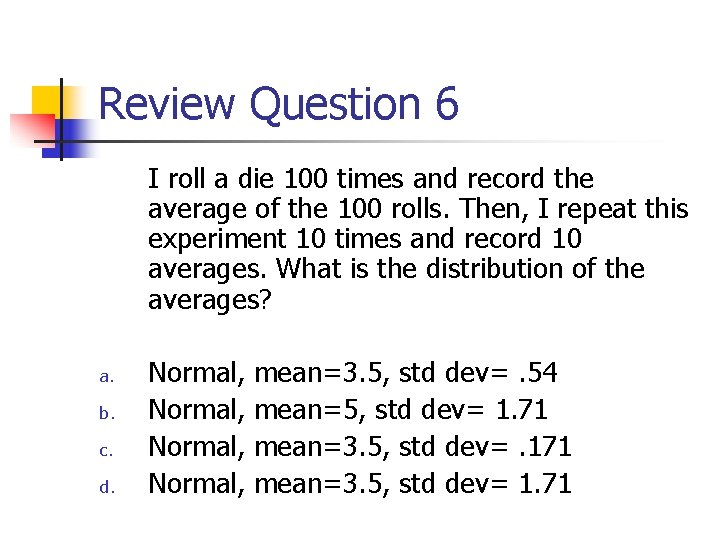Review Question 6 I roll a die 100 times and record the average of the 100 rolls. Then, I repeat this experiment 10 times and record 10 averages. What is the distribution of the averages? a. b. c. d. Normal, mean=3. 5, std dev=. 54 Normal, mean=5, std dev= 1. 71 Normal, mean=3. 5, std dev=. 171 Normal, mean=3. 5, std dev= 1. 71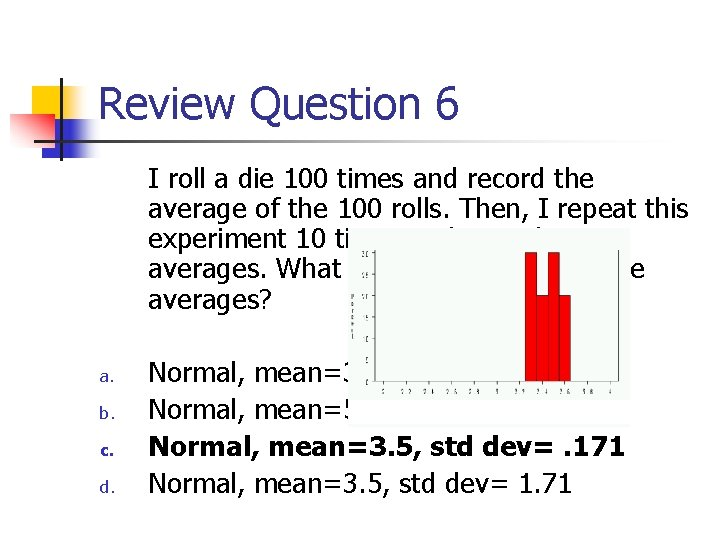Review Question 6 I roll a die 100 times and record the average of the 100 rolls. Then, I repeat this experiment 10 times and record 10 averages. What is the distribution of the averages? a. b. c. d. Normal, mean=3. 5, std dev=. 54 Normal, mean=5, std dev= 1. 71 Normal, mean=3. 5, std dev=. 171 Normal, mean=3. 5, std dev= 1. 71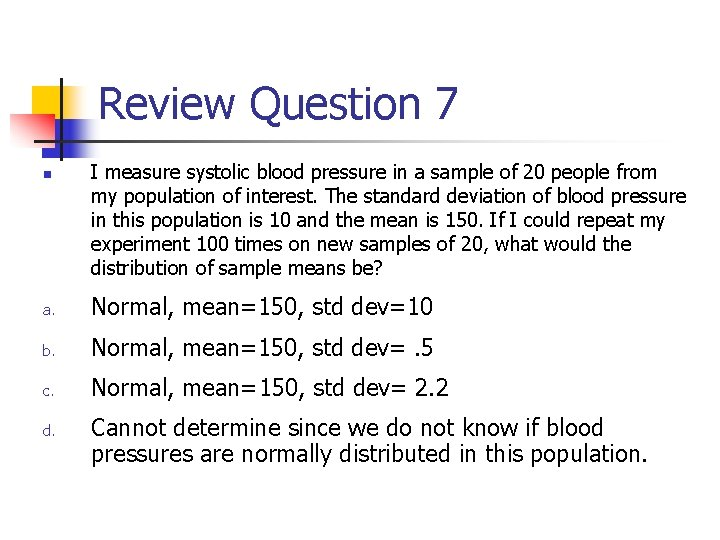Review Question 7 n I measure systolic blood pressure in a sample of 20 people from my population of interest. The standard deviation of blood pressure in this population is 10 and the mean is 150. If I could repeat my experiment 100 times on new samples of 20, what would the distribution of sample means be? a. Normal, mean=150, std dev=10 b. Normal, mean=150, std dev=. 5 c. Normal, mean=150, std dev= 2. 2 d. Cannot determine since we do not know if blood pressures are normally distributed in this population.Review Question 7 n I measure systolic blood pressure in a sample of 20 people from my population of interest. The standard deviation of blood pressure in this population is 10 and the mean is 150. If I could repeat my experiment 100 times on new samples of 20, what would the distribution of sample means be? a. Normal, mean=150, std dev=10 b. Normal, mean=150, std dev=. 5 c. Normal, mean=150, std dev= 2. 2 d. Cannot determine since we do not know if blood pressures are normally distributed in this population.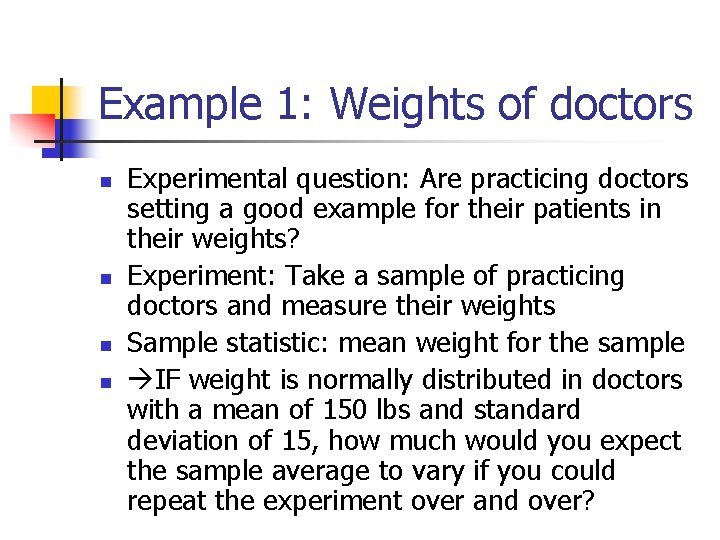Example 1: Weights of doctors n n Experimental question: Are practicing doctors setting a good example for their patients in their weights? Experiment: Take a sample of practicing doctors and measure their weights Sample statistic: mean weight for the sample IF weight is normally distributed in doctors with a mean of 150 lbs and standard deviation of 15, how much would you expect the sample average to vary if you could repeat the experiment over and over?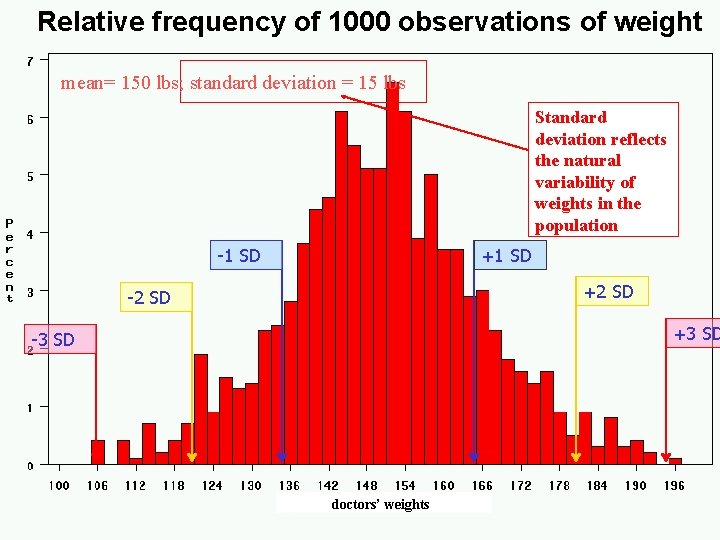Relative frequency of 1000 observations of weight mean= 150 lbs; standard deviation = 15 lbs Standard deviation reflects the natural variability of weights in the population -1 SD +2 SD -2 SD +3 SD -3 SD doctors’ weights-1 SD +2 SD -3 SD +3 SD average 1000 weight doctors’ fromweights samples of 2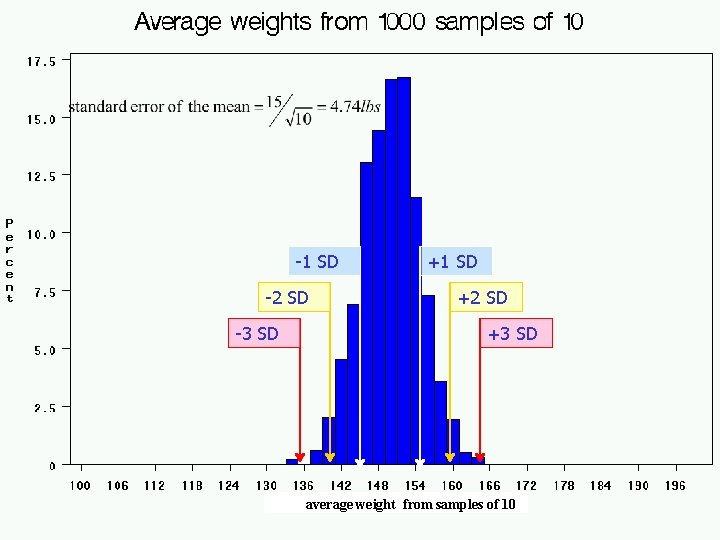-1 SD -2 SD -3 SD +1 SD +2 SD +3 SD average weight from samples of 10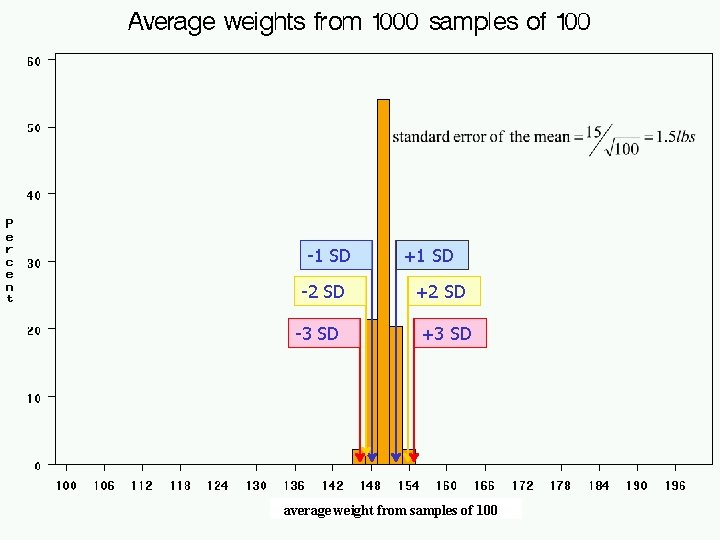-1 SD -2 SD -3 SD +1 SD +2 SD +3 SD average weight from samples of 100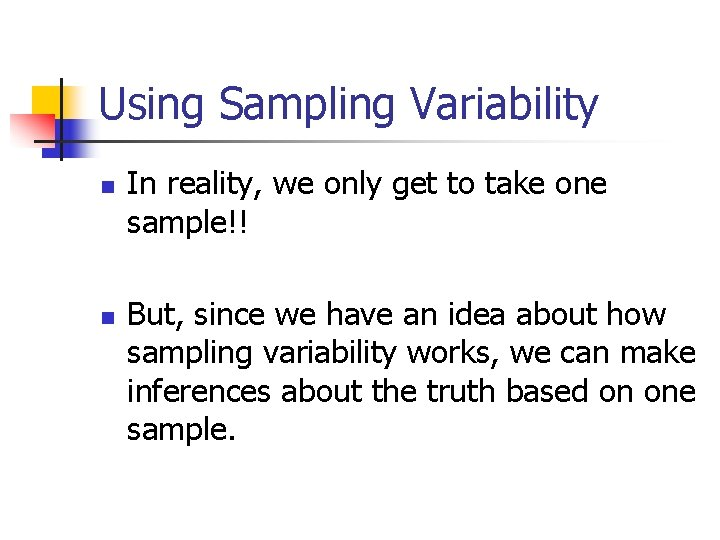Using Sampling Variability n n In reality, we only get to take one sample!! But, since we have an idea about how sampling variability works, we can make inferences about the truth based on one sample.Experimental results n Let’s say we take one sample of 100 doctors and calculate their average weight….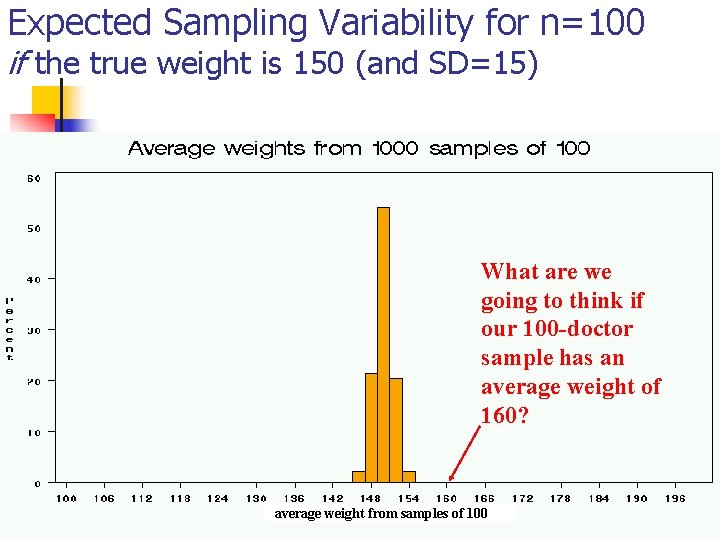Expected Sampling Variability for n=100 if the true weight is 150 (and SD=15) What are we going to think if our 100 -doctor sample has an average weight of 160? average weight from samples of 100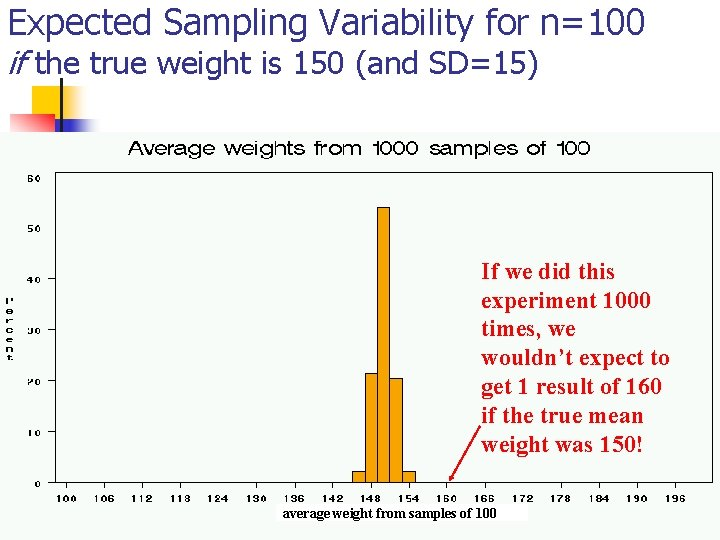Expected Sampling Variability for n=100 if the true weight is 150 (and SD=15) If we did this experiment 1000 times, we wouldn’t expect to get 1 result of 160 if the true mean weight was 150! average weight from samples of 100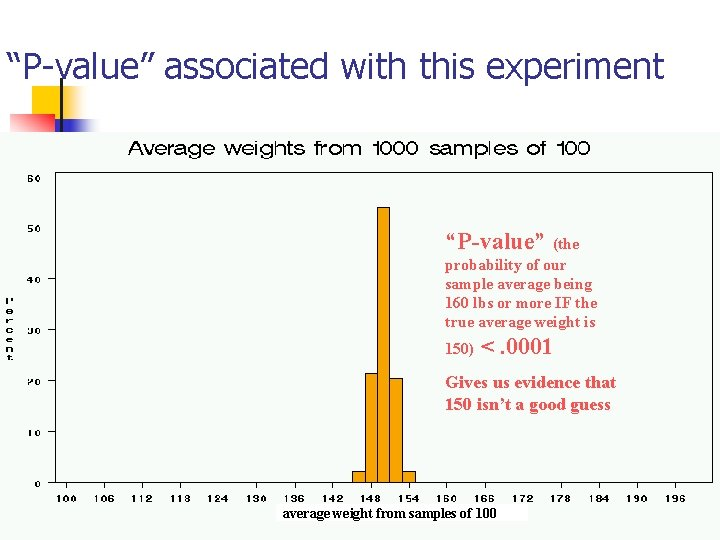“P-value” associated with this experiment “P-value” (the probability of our sample average being 160 lbs or more IF the true average weight is 150) <. 0001 Gives us evidence that 150 isn’t a good guess average weight from samples of 100Calculating the p-value Formally, P-value<. 0001 gives us evidence against our null hypothesis.P-value<. 0001 means: The probability of seeing what you saw or something more extreme if the null hypothesis is true (due to chance)<. 0001 P(empirical data | null hypothesis) <. 0001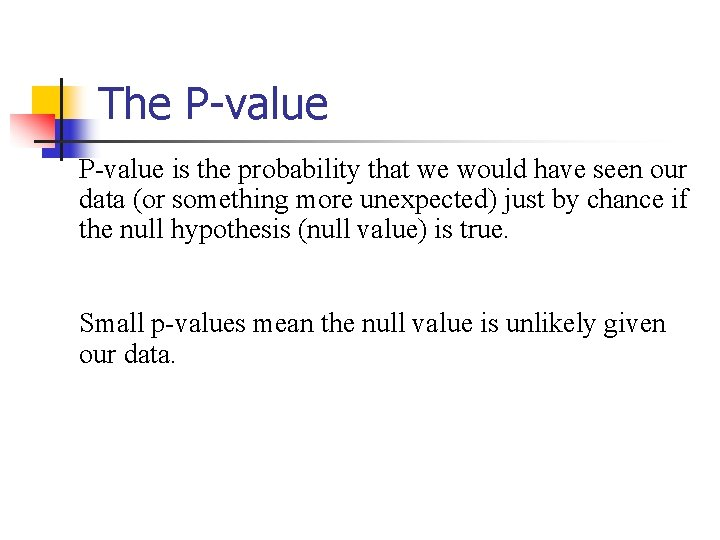The P-value is the probability that we would have seen our data (or something more unexpected) just by chance if the null hypothesis (null value) is true. Small p-values mean the null value is unlikely given our data.The P-value n n By convention, p-values of <. 05 are often accepted as “statistically significant” in the literature; but this is an arbitrary cut-off. A cut-off of p<. 05 means that in about 5 of 100 experiments, a result would appear significant just by chance (“Type I error”).Hypothesis Testing The Steps: 1. Define your hypotheses (null, alternative) 2. Specify your null distribution 3. Do an experiment 4. Calculate the p-value of what you observed 5. Reject or fail to reject (~accept) the null hypothesis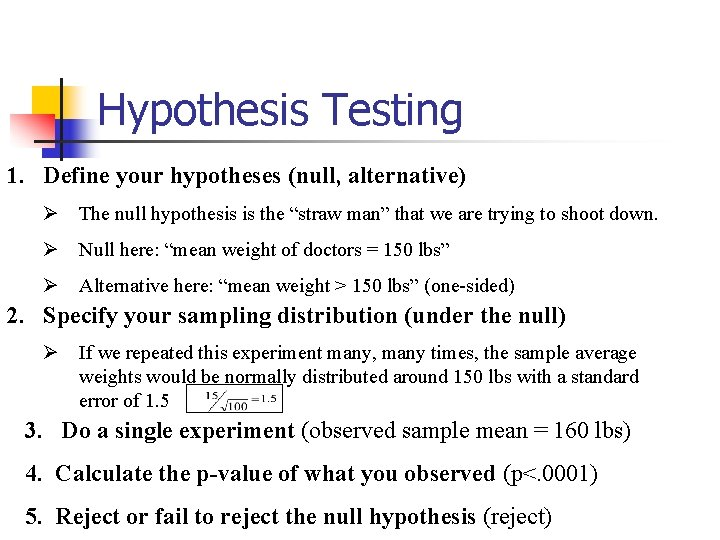Hypothesis Testing 1. Define your hypotheses (null, alternative) Ø The null hypothesis is the “straw man” that we are trying to shoot down. Ø Null here: “mean weight of doctors = 150 lbs” Ø Alternative here: “mean weight > 150 lbs” (one-sided) 2. Specify your sampling distribution (under the null) Ø If we repeated this experiment many, many times, the sample average weights would be normally distributed around 150 lbs with a standard error of 1. 5 3. Do a single experiment (observed sample mean = 160 lbs) 4. Calculate the p-value of what you observed (p<. 0001) 5. Reject or fail to reject the null hypothesis (reject)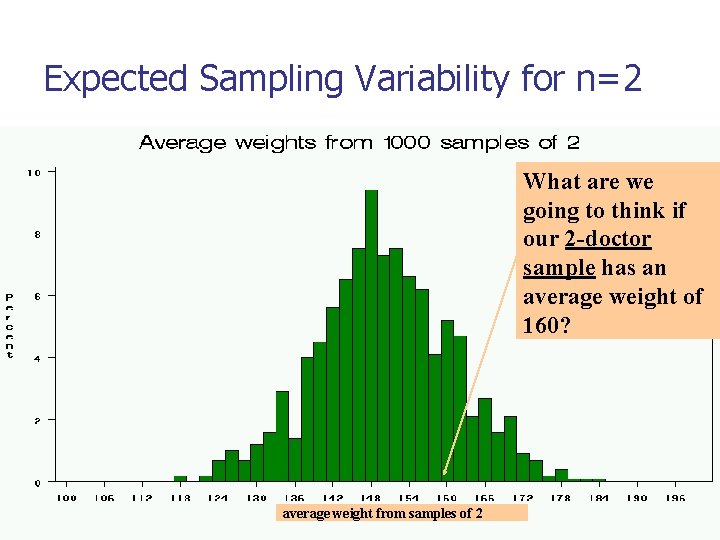Expected Sampling Variability for n=2 What are we going to think if our 2 -doctor sample has an average weight of 160? average weight from samples of 2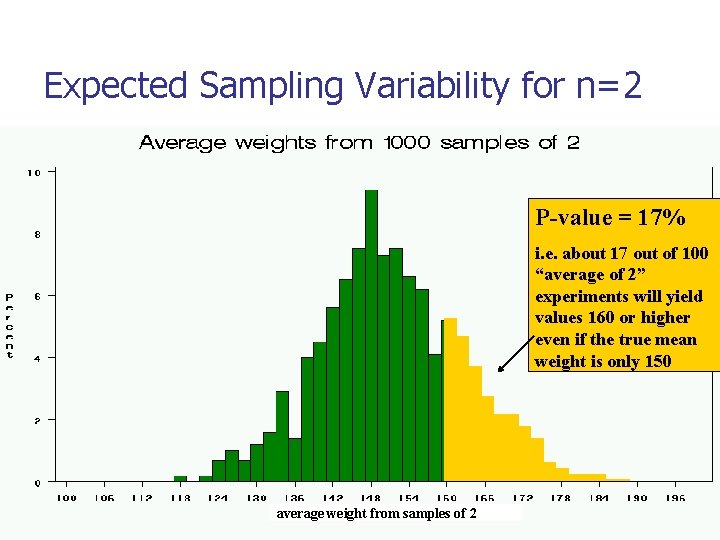Expected Sampling Variability for n=2 P-value = 17% i. e. about 17 out of 100 “average of 2” experiments will yield values 160 or higher even if the true mean weight is only 150 average weight from samples of 2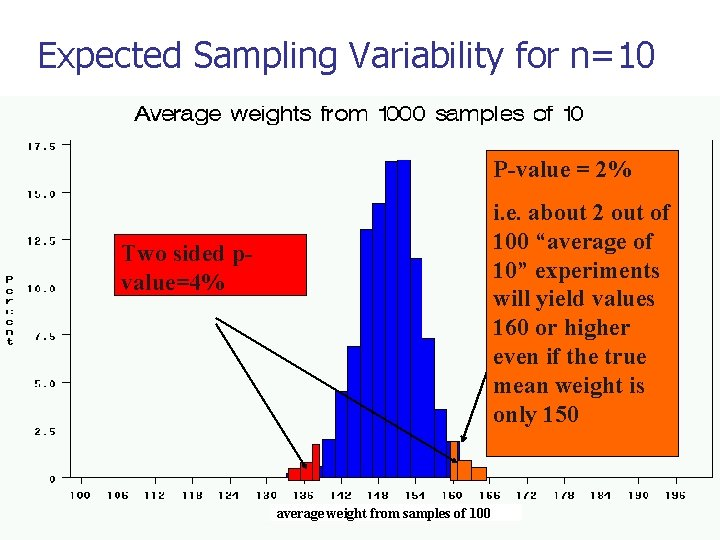Expected Sampling Variability for n=10 P-value = 2% i. e. about 2 out of 100 “average of 10” experiments will yield values 160 or higher even if the true mean weight is only 150 Two sided pvalue=4% average weight from samples of 100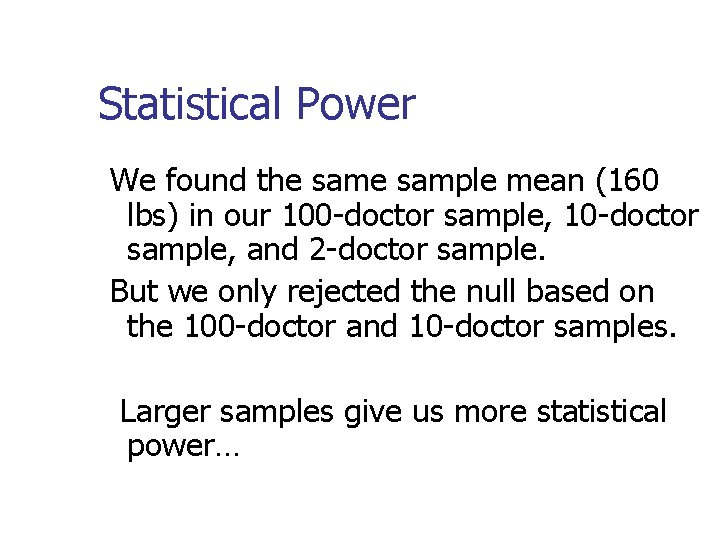Statistical Power We found the sample mean (160 lbs) in our 100 -doctor sample, 10 -doctor sample, and 2 -doctor sample. But we only rejected the null based on the 100 -doctor and 10 -doctor samples. Larger samples give us more statistical power…Hypothesis testing: Error and Power n Type-I Error (also known as “α”): n n Type-II Error (also known as “β “): n n Rejecting the null when the effect isn’t real, e. g. false positive. Failing to reject the null when the effect is real, e. g. false negative. POWER (the flip side of type-II error: 1 - β): n The chance you’ll find an effect if it’s real.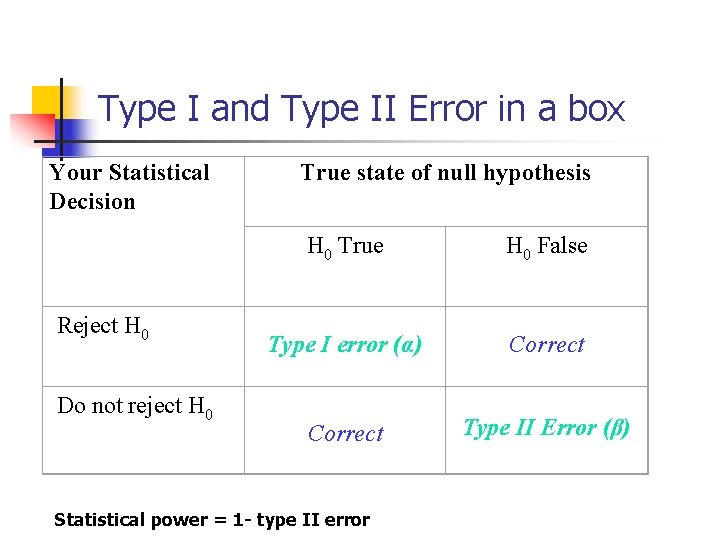Type I and Type II Error in a box Your Statistical Decision Reject H 0 Do not reject H 0 True state of null hypothesis H 0 True H 0 False Type I error (α) Correct Type II Error (β) Statistical power = 1 - type II errorReminds me of… Pascal’s Wager The TRUTH Your Decision Reject God Accept God Exists God Doesn’t Exist BIG MISTAKE Correct— Big Pay Off MINOR MISTAKEReview Question 8 Spine bone density is normally distributed in young women, with a mean of 1. 0 g/cm 2 and a mean of 0. 1 g/cm 2. In my sample of 100 young women runners, the average spine bone density is. 93 g/cm 2. Are runners statistically different from the general population? What is the p-value? a. b. c. d. No, Z=-. 1, p>. 05 Yes, Z=-7, p<. 0001 No, Z=-. 7, p>. 05 Yes, Z=-2, p<. 05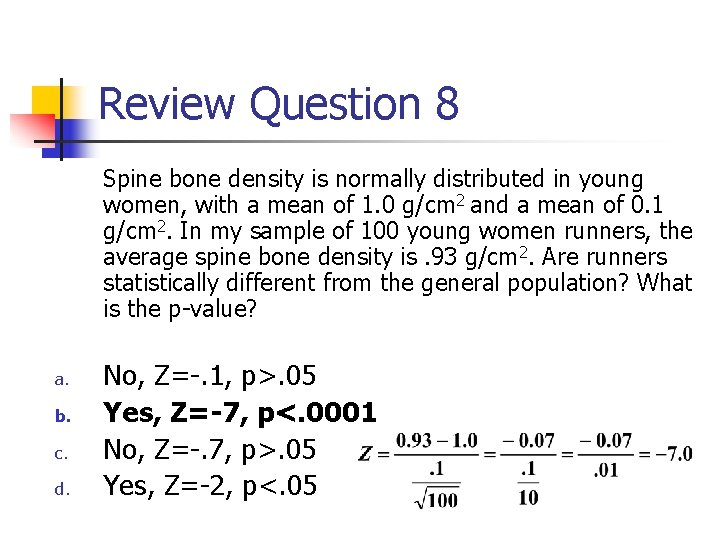Review Question 8 Spine bone density is normally distributed in young women, with a mean of 1. 0 g/cm 2 and a mean of 0. 1 g/cm 2. In my sample of 100 young women runners, the average spine bone density is. 93 g/cm 2. Are runners statistically different from the general population? What is the p-value? a. b. c. d. No, Z=-. 1, p>. 05 Yes, Z=-7, p<. 0001 No, Z=-. 7, p>. 05 Yes, Z=-2, p<. 05Review Question 9 The p-value is: a. b. c. d. The probability that my data are wrong. The probability of my data under the null hypothesis. The probability that I erroneously find an association. The probability that I find an association when one exists.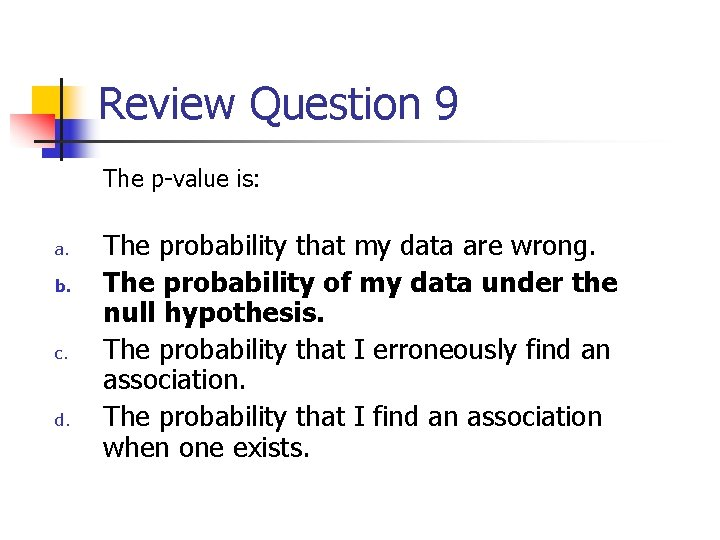Review Question 9 The p-value is: a. b. c. d. The probability that my data are wrong. The probability of my data under the null hypothesis. The probability that I erroneously find an association. The probability that I find an association when one exists.